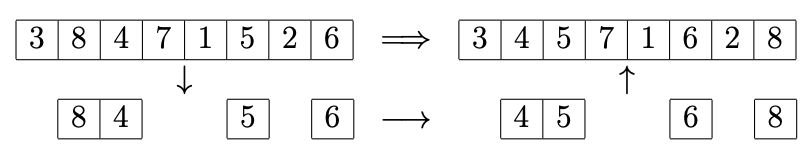시간 제한메모리 제한제출정답맞힌 사람정답 비율
2 초 512 MB126726755.833%

## 문제

Merge sort is a sorting algorithm. It works by splitting an array in half, sorting both halves recursively and then merging those halves together to sort the entire array. Your friend is working on an implementation of the merge sort algorithm, but unfortunately he is not quite there yet: he can only sort half of the array! In great despair he turns to you for help: can you use his unfinished code to write an algorithm that sorts an array completely?

In its current state, your friend's code is a sorting function that can be run on arbitrary sub-arrays, as long as it is precisely half as long as the original array. It then correctly sorts this sub-array. You decide to play around with this function, so you start with a jumbled array and try to sort it (see figure). After choosing $3$ sub-arrays and using them as input for the sorting function, you end up with a sorted array. Interestingly, it seems that no matter what the original array you use is, you can always sort it completely by invoking your friend's sorting function only $3$ times. You decide that this makes for a good challenge: you want to extend the code to work for a full array, making at most three calls to the sorting function.

Now you need to figure out which sub-arrays to sort! Given an array of length $n$, output at most three sub-arrays of length $\tfrac{1}{2}n$ so that sorting these sub-arrays in order will result in a sorted array. It is guaranteed that this is always possible.Figure I.1: First sorting step of sample output 1

## 입력

• One line containing a single integer $n$ ($4\leq n\leq 10^5$) divisible by $4$, the length of the array.
• One line containing $n$ unique integers $a$ ($1\leq a \leq n$), the array to be sorted.

## 출력

The output consists of:

• One line containing the number of function calls $f$ ($0\leq f \leq 3$).
• $f$ lines, each containing $\tfrac{1}{2}n$ unique integers $i$ ($1\leq i \leq n$), the indices determining the sub-array to be sorted at each of the function calls.

If there are multiple valid solutions, you may output any one of them. You do not have to minimise $f$.

## 예제 입력 1

8
3 8 4 7 1 5 2 6


## 예제 출력 1

3
2 3 6 8
1 3 4 5
2 4 5 7


## 예제 입력 2

4
1 4 3 2


## 예제 출력 2

3
3 4
2 3
3 4


## 예제 입력 3

8
1 4 8 7 5 6 3 2


## 예제 출력 3

2
3 5 6 8
2 3 4 7


## 출처• 문제를 만든 사람: Jorke de Vlas